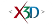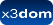## Class: ElevationGrid

X3D: 3.3
Component: Geometry3DExt
Status:
experimental

➨ Fields
➨ Properties

### .nodeTypes. ElevationGrid

The ElevationGrid node specifies a uniform rectangular grid of varying height in the Y=0 plane of the local coordinate system. The geometry is described by a scalar array of height values that specify the height of a surface above each point of the grid. The xDimension and zDimension fields indicate the number of elements of the grid height array in the X and Z directions. Both xDimension and zDimension shall be greater than or equal to zero. If either the xDimension or the zDimension is less than two, the ElevationGrid contains no quadrilaterals. The vertex locations for the rectangles are defined by the height field and the xSpacing and zSpacing fields

### HTML Encoding and Default Values⤴

``` <ElevationGrid attrib='x3dom.nodeTypes.X3DVertexAttributeNode' ccw='true' color='x3dom.nodeTypes.X3DColorNode' colorPerVertex='true' creaseAngle='0' height='[]' lit='true' metadata='X3DMetadataObject' normal='x3dom.nodeTypes.Normal' normalPerVertex='true' solid='true' texCoord='x3dom.nodeTypes.X3DTextureCoordinateNode' useGeoCache='true' xSpacing='1.0' zDimension='0' zSpacing='1.0' ></ElevationGrid> ```

### Constructor⤴

#### ElevationGrid(ctx)⤴

Constructor for ElevationGrid

##### Parameters:
Name Type Argument Default Description
`ctx` Object <optional>
null context object, containing initial settings like namespace

### Fields⤴

These are the X3D / X3DOM fields of this node. Values should usually be received / set as strings via DOM functions (i.e., using `setAttribute("myFieldName", "myFieldValue")` and `getAttribute("myFieldName")`).
Name Type Default Value Range Inheritance Standard Description
attrib MFNode X3DVertexAttributeNodeccw SFBool true X3DGeometryNodeThe ccw field defines the ordering of the vertex coordinates of the geometry with respect to user-given or automatically generated normal vectors used in the lighting model equations.
color SFNode X3DColorNodeThe color field specifies per-vertex or per-quadrilateral colours for the ElevationGrid node depending on the value of colorPerVertex. If the color field is NULL, the ElevationGrid node is rendered with the overall attributes of the Shape node enclosing the ElevationGrid node.
colorPerVertex SFBool trueThe colorPerVertex field determines whether colours specified in the color field are applied to each vertex or each quadrilateral of the ElevationGrid node. If colorPerVertex is FALSE and the color field is not NULL, the color field shall specify a node derived from X3DColorNode containing at least (xDimension-1)×(zDimension-1) colours; one for each quadrilateral. If colorPerVertex is TRUE and the color field is not NULL, the color field shall specify a node derived from X3DColorNode containing at least xDimension × zDimension colours, one for each vertex.
creaseAngle SFFloat 0 [0, inf]The creaseAngle field affects how default normals are generated. If the angle between the geometric normals of two adjacent faces is less than the crease angle, normals shall be calculated so that the faces are shaded smoothly across the edge; otherwise, normals shall be calculated so that a lighting discontinuity across the edge is produced. Crease angles shall be greater than or equal to 0.0 angle base units.
height MFFloat []The height field is an xDimension by zDimension array of scalar values representing the height above the grid for each vertex.
lit SFBool true X3DGeometryNodeSpecifies whether this geometry should be rendered with or without lighting.
metadata SFNode X3DMetadataObject X3DNodeField to add metadata information
normal SFNode NormalThe normal field specifies per-vertex or per-quadrilateral normals for the ElevationGrid node. If the normal field is NULL, the browser shall automatically generate normals, using the creaseAngle field to determine if and how normals are smoothed across the surface.
normalPerVertex SFBool trueThe normalPerVertex field determines whether normals are applied to each vertex or each quadrilateral of the ElevationGrid node depending on the value of normalPerVertex. If normalPerVertex is FALSE and the normal node is not NULL, the normal field shall specify a node derived from X3DNormalNode containing at least (xDimension−1)×(zDimension−1) normals; one for each quadrilateral. If normalPerVertex is TRUE and the normal field is not NULL, the normal field shall specify a node derived from X3DNormalNode containing at least xDimension × zDimension normals; one for each vertex.
solid SFBool true X3DGeometryNodeSpecifies whether backface-culling is used. If solid is TRUE only front-faces are drawn.
texCoord SFNode X3DTextureCoordinateNodeThe texCoord field specifies per-vertex texture coordinates for the ElevationGrid node. If texCoord is NULL, default texture coordinates are applied to the geometry.
useGeoCache SFBool true X3DGeometryNodeMost geo primitives use geo cache and others might later on, but one should be able to disable cache per geometry node. Defaults to global useGeoCache setting parameter.
xSpacing SFDouble 0 [0, inf]zDimension SFInt32 0 [0, inf]Defines the grid size in z.
zSpacing SFDouble 0 [0, inf]